Courses

# Structural Fasteners - 3

## 20 Questions MCQ Test Topicwise Question Bank for GATE Civil Engineering | Structural Fasteners - 3

Description
This mock test of Structural Fasteners - 3 for Civil Engineering (CE) helps you for every Civil Engineering (CE) entrance exam. This contains 20 Multiple Choice Questions for Civil Engineering (CE) Structural Fasteners - 3 (mcq) to study with solutions a complete question bank. The solved questions answers in this Structural Fasteners - 3 quiz give you a good mix of easy questions and tough questions. Civil Engineering (CE) students definitely take this Structural Fasteners - 3 exercise for a better result in the exam. You can find other Structural Fasteners - 3 extra questions, long questions & short questions for Civil Engineering (CE) on EduRev as well by searching above.
QUESTION: 1

Solution:
QUESTION: 2

Solution:
QUESTION: 3

### When load line coincides with the C.G. of rivet group, then the rivets are subjected to

Solution:

When a load, W acts on a rivet group then the rivets are subjected to two forces,
1. Force due to axial load, Fa = W/n
n = total number of rivets
2. Force due to moment M on any rivet Fm ∞ r
r = distance of the rivet from the C.G. of the rivet group
when load line coincides with the C.G. of the rivet group i.e. non-eccentric connection then the rivets are subjected to shear only.

QUESTION: 4

For reversal of stresses the most suitable bolt is

Solution:

Friction grip bolt is also called. High strength friction grip bolt or HSFG. The fatigue strength of these bolts is more because of no stress concentrations in the holes which makes it suitable to withstand reversal of stresses.

QUESTION: 5

Which of the following is in violation of assumptions made in riveted joint analysis

Solution:
QUESTION: 6

If the same number of rivets has been used in the joints then which of the following pattern will yield highest efficiency

Solution:

The width of the main plate required is less in case diamond riveting. The critical section is diamond riveting passes through one or two rivet holes. The width of the plate required to accommodate diamond pattern is less than that for chain pattern by d(n - 1).
n = Number of rivets in chain riveting at critical section
d = Gross diameter of rivets

QUESTION: 7

Minimum pitch of rivets in a riveted joint is

Solution:

Minimum pitch = 2.5 times the nomial dia of rivet.

QUESTION: 8

The effective length of a fillet weld of length L and size s is given by

Solution:

The effective length of a fillet weld is equal to its overall length minus twice the weld size. The effective length of a fillet weld designed to transmit loading should not be. less than four times weld size.

QUESTION: 9

The overlap of batten plates with the main members in welded connections should be more than (where t = thickness of batten plate)

Solution:
QUESTION: 10

For a standard 45° fillet weld the ratio of size of fillet to throat thickness is

Solution:

For a 45° fillet weld throat thickness t = √2 x size of the weld.

QUESTION: 11

The common assumption that, ‘all rivets share equally a non-eccentric load’ is valid at a load

Solution:

Actually the outer rivets are subjected to greater shear as compared to the inner rivets. The assumption that all rivets share equally a non-eccentric load is approximately valid when the connection is subjected to static loads approaching ultimate strength.

QUESTION: 12

By providing sufficient edge distance, which of the following failures of riveted joint can be avoided?

Solution:
QUESTION: 13

As per IS : 800, the rivets subjected to combined tensile and shear stresses are proportioned such that
(Where fs and ft are respectively actual shear and tensile stresses in a rivet and ps and pt are respectively permissible shear and tensile stresses in rivet.)

Solution:
QUESTION: 14

In a diamond riveting, for a plate of width 'b' and rivet diameter 'd', the efficiency of the joint is given by

Solution:

Efficiency of riveted joint,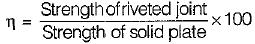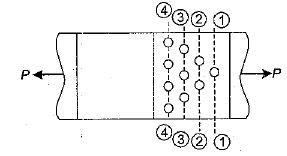The section 1 - 1 is most critical. The strength of joint at section 1 - 1 is (b - d)tσat.
The strength of solid plate is b tσat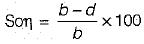QUESTION: 15

Which of the following methods of design would be suitable for metal structures subjected to stress reversals and impact?
1. Simple working stress design
2. Rigid plastic design
3. Semirigid design
4. Elastic rigid design

Select the correct answer using the codes given below:

Solution:
QUESTION: 16

For rivets in tension with countersunk heads, the tensile value shall be

Solution:
QUESTION: 17

When two plates are placed end to end and are joined by two cover plates the joint is known is

Solution:
QUESTION: 18

Match List - l (Use) with List - ll (Type of weld) and select the correct answer using the codes given below the lists:
List - l
A. Structural members subject to direct tension or compression
B. Joining two surfaces approximately at right angIes to each other
C. A hole is made in one of the components and welding is done around the periphery of the hole.
D. Pressure is applied continuously
List - ll
1. Slot weld
2. Seam weld
3. Fillet weld
4. Plug weld
5. Butt weld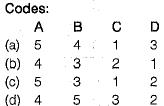Solution:
QUESTION: 19

Consider the following statements:
Aluminum is being increasingly used for structural purposes because
1. Its modulus of elasticity is double that of steel
2. Its coefficient of thermal expansion is half that of steel
3. It requires less maintenance
4. The strength to unit weight ratio of aluminum is high

Which of these statements are correct?

Solution:
QUESTION: 20

The rivets in an eccentrically loaded riveted joint are shown in figure below.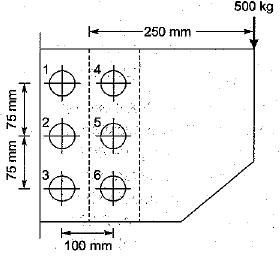Which rivets will be stressed to maximum?

Solution: